Latest SSC jobs   »   Mixture and Alligation

# Mixture and Alligation, Notes, Methods and Questions

## Mixture and Alligation

Mixture and Alligation: Quantitative Aptitude is a very important section for government recruitment exams like SSC, railway, and banks. In this article, we are going to discuss the rule of mixtures and allegations. Generally, there are questions asked related to basic concepts and formulas of the Mixture and Alligation. The rule of alligation enables us to find the ratio in which two or more ingredients at the given price must be mixed to produce a mixture of the desired price. We have covered important notes and questions focusing govt. exams that aspirants can practice and prepare for the mixture and alligation.

Mixture: An aggregate of two or more two types of quantities gives us a mixture.

Alligation: It is a method of solving arithmetic problems related to mixtures of ingredients. This rule enables us to find the ratio in which two or more ingredients at the given price must be mixed to produce a mixture of the desired price.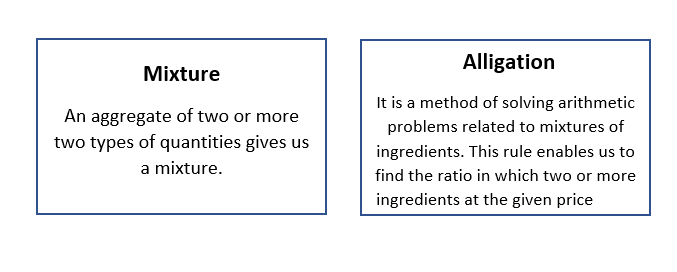### Mixture and Alligation Formula

• It is a modified form of finding the weighted average. If 2 ingredients are mixed in a ratio and the cost price of the unit quantity of the mixture, called the Mean Price is given then,The above formula can be represented with the help of a diagram which is easier to understand. Here ‘d’ is the cost of a dearer ingredient, ‘m’ is mean price and ‘c’ is the cost of cheaper ingredient.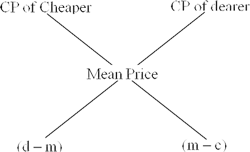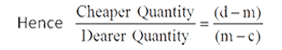Thus, (Cheaper quantity) : (Dearer quantity) = (d – m) : (m – c).

### Mixture and Alligation Formula: Repeated Dilution

• This is used to calculate the pure quantity left after ‘n’ number of processes of repeated replacement is done on the pure quantity. Suppose, a container contains ‘x’ units of a liquid from which ‘y’ units are taken out and replaced by water. After ‘n’ operations quantity of pure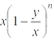Click Here to Attempt More Questions From Quantitative Aptitude For SSC CGL

### Mixture and Alligation Tricks

The competitive exam is totally based on time management to solve the questions with accuracy. Time management is very important to score more and attempt more questions in less time. Some mixture and allegation tips and tricks are very helpful for solving the word problems in a few minutes. Candidates must try to solve the problems using the tricks. Candidates must note that any tip or trick will be useful only if the candidate practices it a lot.

### Mixture and Alligation Questions

1. A container contains 40 liters of milk. From this container, 4 liters of milk were taken out and replaced by water. This process was repeated further two times. How much milk is now contained in the container?

A. 26 liters
B. 29.16 liters
C. 28 liters
D. 28.2 litres
2. A vessel is filled with liquid, 3 parts of which are water and 5 parts syrup. How much of the mixture must be drawn off and replaced with water so that the mixture may be half water and half syrup?
A. 1/3
B. 1/4
C. 1/5
D. 1/7
3. Tea worth Rs. 126 per kg and Rs. 135 per kg are mixed with a third variety of tea in the ratio of 1 : 1: 2. If the mixture is worth Rs. 153 per kg, what is the price of the third variety per kg?
A. Rs.182.50
B. Rs.170.5
C. Rs.175.50
D. Rs.180
4. A can contain a mixture of two liquids A and B is the ratio of 7: 5. When 9 liters of the mixture are drawn off and the can is filled with B, the ratio of A and B becomes 7: 9. How many liters of liquid A were contained in the can initially?
A. 10
B. 20
C. 21
D. 25
5. Two vessels A and B contain spirit and water in the ratio of 5: 2 and 7: 6 respectively. Find the ratio in which these mixtures be mixed to obtain a new mixture in vessel C containing spirit and water in the ratio 8: 5?
A. 3: 4
B. 4 : 3
C. 9: 7
D. 7: 9
6. In what ratio must a grocer mix two varieties of pulses costing Rs. 15 and Rs. 20 per kg respectively so as to get a mixture worth Rs. 16.50 kg?
A. 3: 7
B. 5: 7
C. 7 : 3
D. 7: 5
7.8 liters are drawn from a cask full of wine and are then filled with water. This operation is performed three more times. The ratio of the quantity of wine now left in the cask to that of the water is 16: 65. How much wine did the cask originally hold?
A. 30 liters
B. 26 liters
C. 24 litres
D. 32 litres
8. A dishonest milkman professes to sell his milk at cost price but he mixes it with water and thereby gains 25%. The percentage of water in the mixture is:
A. 4%
B. 6 1/4%
C. 20%
D. 25%
9. A jar full of whiskey contains 40% alcohol. A part of this whisky is replaced by another containing 19% alcohol and now the percentage of alcohol was found to be 26%. The quantity of whisky replaced is
A. 4/3
B. 3/4
C. 3/2
D. 2/4

### Mixture and Alligation Answers & Solutions

1( B
Explanation
Assume that a container contains x of liquid from which y units are taken out and replaced by water. After n operations, the quantity of pure liquid
=x(1-y/x)^n
Hence milk now contained by the container = 40(1-4/40)^3
=40(1-1/10)^3
=40×9/10×9/10×9/10 =(4×9×9×9)/100 =29.16
2(C)
Explanation
Suppose the vessel initially contains 8 litres of liquid.
Let x litres of this liquid be replaced with water.
Quantity of water in new mixture = (3 – 3x/8 + x) litre
Quantity of syrup in new mixture = (5 – 5x/8) litres
So  (3 – 3x/8 + x)  = (5 – 5x/8) litres
=> 5x + 24 = 40 – 5x
=>10x = 16
=> x = 8/5 .
So, part of the mixture replaced = (8/5 x 1/8) = 1/5
3(C)
Explanation :
Tea worth Rs. 126 per kg and Rs. 135 per kg are mixed in the ratio 1 : 1
So their average price = (126+135)/2=130.5
Hence let’s consider that the mixture is formed by mixing two varieties of tea.
one at Rs. 130.50 per kg and the other at Rs. x per kg in the ratio 2 : 2, i.e., 1 : 1. Now let’s find out x.
By the rule of alligation, we can write asCost of 1 kg of 1st kind of tea              Cost of 1 kg of 2nd kind of tea
(130.50)                                                     (x)Mean Price
(153)(x – 153)                                                                     (22.50)=>(x – 153) : 22.5 = 1 : 1
=>x – 153 = 22.50
=> x = 153 + 22.5 = 175.54(C)Explanation:
Suppose the can initially contains 7x and 5x of mixtures A and B respectively.
Quantity of A in mixture left = (7x – 7/12 x 9)litres
= (7x – 21/4) litres.
Quantity of B in mixture left = (5x – 5/12  x 9) litres
= (5x – 15/4)  litres.
So (7x – 21/4)/((5x – 15/4) +9) = 7/9
=> (28x – 21)/(20x + 21) = 7/9
=> 252x – 189 = 140x + 147
=> 112x = 336
=> x = 3.
So, they can contain 21 liters of A.5(D)
Explanation :
Let the Cost Price(CP) of a 1-liter spirit be Rs.1
Quantity of spirit in 1-liter mixture from vessel A = 5/7
Cost Price(CP) of 1 litre mixture from vessel A = Rs. 5/7
Quantity of spirit in 1-liter mixture from vessel B = 7/13
Cost Price(CP) of 1 litre mixture from vessel B = Rs. 7/13
Quantity of spirit to be obtained in 1 liter mixture from vessel C = 8/13
Cost Price(CP) of 1 litre mixture from vessel C = Rs. 8/13 = Mean Price
By the rule of alligation, we can write asCP of 1 liter mixture     CP of 1 liter mixture
from vessel A (5/7)      from vessel B (7/13)
Mean Price
(8/13)8/13 – 7/13 = 1/13                 5/7 – 8/13 = 9/91=> Mixture from Vessel A : Mixture from Vessel B = 1/13 : 9/91 = 7 : 9 = Required Ratio

6(C)
Explanation:
By the rule of alligation:
Cost of 1 kg pulses of       Cost of 1 kg pulses of
1st kind Rs.  (15)               2nd kind Rs.(20)

Mean Price
Rs. (16.50)

(3.50)                                    (1.50)

Required rate = 3.50 : 1.50 = 7 : 3.

7(C)
Explanation :
Let initial quantity of wine = x litre
After a total of 4 operations, quantity of wine = x(1-y/x)^n=x(1-8/x)^4
Given that after a total of 4 operations, the ratio of the quantity of wine left in cask to that of water = 16 : 65
Hence we can write as (x(1-8/x)^4)/x =16/81
(1-8/x)^4 = (2/3)^4
(1-8/x) = 2/3
(x-8/x) = 2/3
3x-24=2x
x=24

8(C)
Explanation:
Let C.P. of 1 liter milk be Re. 1
Then, S.P. of 1 liter of mixture = Re. 1, Gain = 25%.
C.P. of 1 litre mixture = Re.(100/125 x 1) = 4/5
By the rule of alligation, we have:

C.P. of 1 liter of milk       C.P. of 1 liter of water
Re. (1)                                     (0)

Mean Price
Re. 4/5

4/5                                       1/5

Ratio of milk to water = 4/5 : 1/5 = 4 : 1.
Hence, percentage of water in the mixture = (1/5 x 100)% = 20%

9(D)
Explanation :
The concentration of alcohol in 1st Jar = 40%
The concentration of alcohol in the 2nd Jar = 19%
After the mixing, the concentration of alcohol in the mixture = 26%
By the rule of alligation,
The concentration of alcohol in
1st Jar(40%)                                 2nd Jar (19%)

Mean
(26%)

(7)                                             (14)

Hence ratio of 1st and 2nd quantities = 7 : 14 = 1 : 2

## Mixture and Alligation: FAQ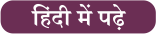#### Congratulations!General Awareness & Science Capsule PDFIncorrect details? Fill the form again here

General Awareness & Science Capsule PDF

Thank You, Your details have been submitted we will get back to you.
•Time and Work Notes, Simple Tricks To So...
•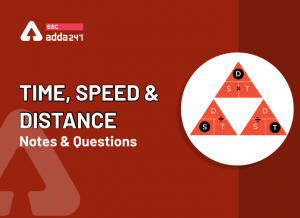Speed, Time And Distance Tricks and Ques...
•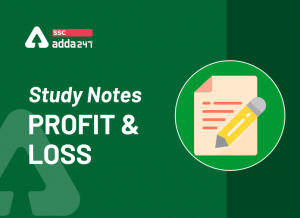Profit and Loss Formula, Concept and Stu...
•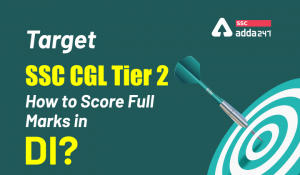Target SSC CGL Tier 2: How to Score Full...
•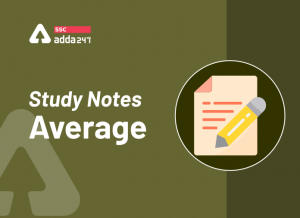Study Notes On Average Formula With Exam...
•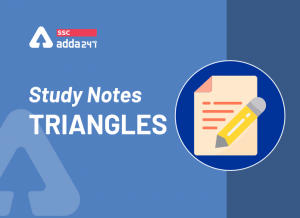Triangles: Notes and Questions

Join the Conversation

1.1.BÃRBÎÊ GÎRL says:

@Mosaic

2.Pallavi Shekar_DREAM BIG :D says:

_/_ 🙂

3.kumar says:

Thank u sir,,very useful

4.Prabhas says:

1.B

5.Gladiator:: The Bibliophile :) says:

..

6.BÃRBÎÊ GÎRL says:

Gladi maths k post pe aa jaya kro discuss krne

7.L@mbkin_SBI clerk says:

solve krne mei baht problem h abhi bhi 🙁

8.BÃRBÎÊ GÎRL says:

I hate dis chapter,bt will try

9.Säuräbh~ {ʍ€gąʍɨɲď} says:

..

10.BÃRBÎÊ GÎRL says:

Aap yaha bhi:))

11.Prabhas says:

2.B ?

12.Säuräbh~ {ʍ€gąʍɨɲď} says:

jaha mam bulaenge waha ham jaenge 😛

13.BÃRBÎÊ GÎRL says:

Hihi :)) mega kuch tips de so sbi or Po k liye is baar clear ho jaye

14.L@mbkin_SBI clerk says:

1=b
3=c

15.Säuräbh~ {ʍ€gąʍɨɲď} says:

ye jo gladiator ji dikh rhe haina.. ye abhi medical clear karwake aye hai.. direct inhi se tips lelo.. hm bhi inse he lete hain 😀

16.BÃRBÎÊ GÎRL says:

Gladi kaha ho. Its me Sam

17.BÃRBÎÊ GÎRL says:

Hanji jaroor 🙂

18.Mjjoshi says:

1-b ,2-c ,3-c

19.Benaam Shayar says:

:O mixture n alligation _/_
lunch k baad try krta hoon..:P

20.BÃRBÎÊ GÎRL says:

Mt kro lunch

21.Benaam Shayar says:

😛 ammmma bhuk lag gyi

22.BÃRBÎÊ GÎRL says:

Shayar yar ye chapter samajh me aata kabhi bhi plz help.after Lunch

23.Benaam Shayar says:

ok.. lunch k baad aata hoon..
tab tak try krte rhiyo

24.Säuräbh~ {ʍ€gąʍɨɲď} says:
25.BÃRBÎÊ GÎRL says:

Hmm..

26.gusto says:

B.B.C.C.?.C.C.C.D.B

27.Mjjoshi says:

explain ???

28.angela says:

????..

29.angela says:

explain..?

30.Säuräbh~ {ʍ€gąʍɨɲď} says:

which one

31.Mjjoshi says:

4-10 pls explain??

32.Ethan hunt says:

B,?,C,C,D,C,C,C,D,B

33.gusto says:

Let 100 ml was there and it contained 40 alcohol
No 40(1-x)+19*x= 26
X= 2/3

34.Säuräbh~ {ʍ€gąʍɨɲď} says:

saare..
wait answers explanations ke sth ajaenge

35.angela says:

any problem.. i hav problem dis chapter

36.BÃRBÎÊ GÎRL says:

Without explanation :((

37.Ethan hunt says:

Explain to mam krengi naaa

38.BÃRBÎÊ GÎRL says:

Han…

39.BÃRBÎÊ GÎRL says:

Aajkal quiz ne hoti kya yaha @mosaic ki??

40.Ethan hunt says:

dikha nhi..mujhe kal se vo……

41.BÃRBÎÊ GÎRL says:

Han mai bhi usey hi search kar rahi hu kal se.. 🙁

42.ratnab. says:

Bhai log SSC cgl me challan banvane ke bad kya krna hoga.pic and sign phle hi upload ho gya hai

43.@wari!!* says:
44.Rohit chauhan says:

D most easiest one

45.Rohit chauhan says:

D most easiest one

46.Satish says:

Hi, after the payment step of SSC CGL application I didnt get any email or msg confirmation from SSC. Is this happening with everyone?????

47.freedomfighter says:

ur from which region?

48.Gladiator:: The Bibliophile :) says:

Uff…..sam…
Pehchan hi nahi saka…
Hi sam …hw r u??

49.BÃRBÎÊ GÎRL says:

Hello,,gladi yr mujhe sbi po and ibps po k liye tips do,,I wanna clear exam this year abhi tk 1single paper clear ne hua hai almost 1 1/2 yr ho gaye hai

50.Dhruba Jyoti Thakuria says:

Why in questions no. 2 it is multipled with 1/8. Please explain

51.Viveksingh Chauhan says:

Dear admin please suggest me in question no 7 the value is 16/81 or 16/65 you mention here 16/65 please explain

52.Gaurav says:

Same doubt…what happened in que no 7

53.Divik Shah says:

Plz explain 2 & 4 questions. …

54.priyanshu says:

Bcz of in qustion mention, ratio(16:65) is obtain after drawn liquid so when we equate this then we take total quantity of liqued(16/16+65)=16:81..

55.Viveksingh Chauhan says:

Thanks a lot priyanshu ji for clear my doubt

56.Mahi Walia says:

Yaar ye Slov kase kaiya h samja hi nhi aa rha

57.Mahi Walia says:

Post office. Ka exam h